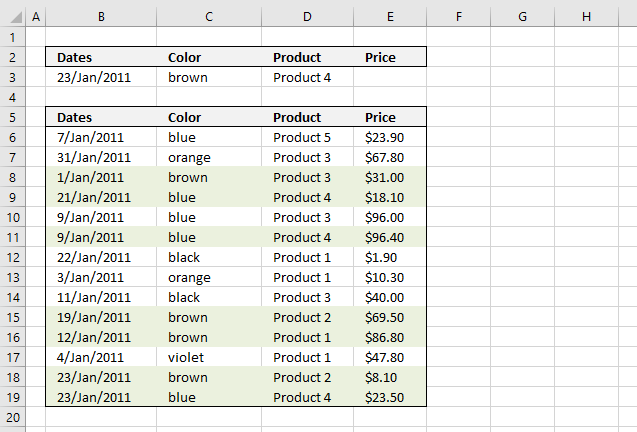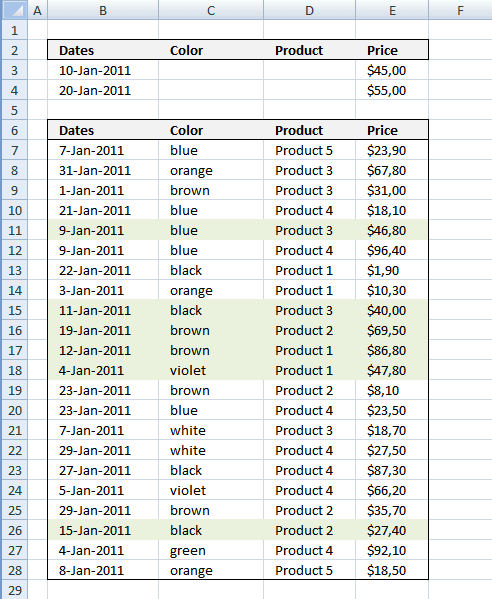Author: Oscar Cronquist Article last updated on January 14, 2019

###The image above shows you how to highlight rows with multiple criteria using OR logic. The criteria are found in row 3.

A row is highlighted if:

• Date criterion is found in column B or
• Color criterion is found in column C or
• Product criterion is found in column D or
• Price criterion is found in column E

### Conditional formatting formula:

=SUMPRODUCT((\$B\$3=\$B6)+(\$C\$3=\$C6)+(\$D\$3=\$D6)+(\$E\$3=\$E6))

### Explaining formula in cell D11

Step 1 - Understand how relative and absolute cell references work

In cell B6 the formula is: =SUMPRODUCT((\$B\$3=\$B6)+(\$C\$3=\$C6)+(\$D\$3=\$D6)+(\$E\$3=\$E6))

and in cell D11 the formula has changed to =SUMPRODUCT((\$B\$3=\$B11)+(\$C\$3=\$C11)+(\$D\$3=\$D11)+(\$E\$3=\$E11))

Step 2 - OR logic is created using + signs between criteria

=SUMPRODUCT((\$B\$3=\$B11)+(\$C\$3=\$C11)+(\$D\$3=\$D11)+(\$E\$3=\$E11))

becomes

=SUMPRODUCT((40566=40552)+("brown"="blue")+("Product 4"="Product 4")+(""=96,40))

becomes

=SUMPRODUCT(FALSE+FALSE+TRUE+FALSE)

becomes

=SUMPRODUCT(0+0+1+0) and returns 1. (TRUE)

Cell D11 is highlighted

### How to apply conditional formatting formula

1. Select cells B6:E27
2. Click "Home" tab
3. Click "Conditional Formatting" button
4. Click "New Rule.."
5. Click "Use a formula to determine which cells to format"
6. Type =SUMPRODUCT((\$B\$3=\$B6)+(\$C\$3=\$C6)+(\$D\$3=\$D6)+(\$E\$3=\$E6)) in "Format values where this formula is TRUE" window.
7. Click "Format.." button
8. Click "Fill" tab
9. Select a color for highlighted cells.
10. Click "Ok"
11. Click "Ok"
12. Click "Ok"
Note: Conditional formatting is volatile meaning when the worksheet is recalculated all conditionally formatted cells are recalculated, this may slow down your worksheet considerably.

### Date range and price range criteria

Let's set up a new sheet with a date range as a criterion and a price range as another criterion.

A row is highlighted if:

• A date is smaller or equal to cell B4 and the same date is larger or equal to cell B3 or
• Color criterion is found in column C or
• Product criterion is found in column D or
• A price is smaller or equal to cell E4 and the same price is larger or equal to cell E3### Conditional formatting formula:

=SUMPRODUCT((\$B\$3<=\$B7)*(\$B\$4>=\$B7)+(\$C\$3=\$C7)+(\$D\$3=\$D7)+(\$E\$3<=\$E7)*(\$E\$4>=\$E7))

### How to apply conditional formatting formula

1. Select cells B6:E27
2. Click "Home" tab
3. Click "Conditional Formatting" button
4. Click "New Rule.."
5. Click "Use a formula to determine which cells to format"
6. Type =SUMPRODUCT((\$B\$3<=\$B7)*(\$B\$4>=\$B7)+(\$C\$3=\$C7)+(\$D\$3=\$D7)+(\$E\$3<=\$E7)*(\$E\$4>=\$E7)) in "Format values where this formula is TRUE" window.
7. Click "Format.." button
8. Click "Fill" tab
9. Select a color for highlighted cells.
10. Click "Ok"
11. Click "Ok"
12. Click "Ok"